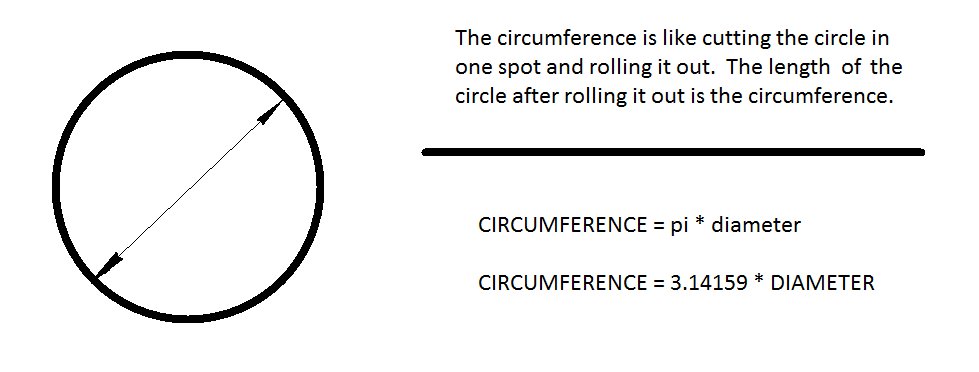# How to Calculate Your Robot’s Speed or Velocity

Calculating the speed of your robot creations is a basic skill for good robot building.  I hope the below tutorial helps you to pick out the correct gear ratio for your motor and wheel size for your robots.

Step 1)

In order calculate the speed of your wheel driven robot, we first need to review some basic geometry.As you keep the larger diameter you choose for robot, the further the robot will travel in one rotation of its wheels.  For instance, if you pick 3″ diameter wheels, the circumference is 3.14159 times 3 which equates to 9.42 inches.  With each rotation (assuming no wheel slippage) of the wheel your robot would travel 9.42 inches using 3 inch diameter wheels.  OK, let’s do the calculation one more time and this time choose 4″ diameter wheels.  The circumference would equate to 3.14159 * 4 or 12.57 inches.  As you see, choosing large wheels should equate to higher speeds since each rotation propels your robot further.

So how do we use this simple geometry to calculate the theoretical speed of our robots?

The answer comes from a simple formulas to calculate the speed:

FPM = Cf * WRPM (wheel RPM * circumference in feet = feet per minute)
FPM * 60 = FPH (feet per minute * 60 minutes = feet per hour)
FPH / 5280 = MPH (feet per hour / 5280 feet = miles per hour)